# 10.8 Vectors  (Page 11/22)

 Page 11 / 22

For the following exercises, evaluate each root.

Evaluate the cube root of $\text{\hspace{0.17em}}z\text{\hspace{0.17em}}$ when $\text{\hspace{0.17em}}z=64\mathrm{cis}\left(210°\right).$

Evaluate the square root of $\text{\hspace{0.17em}}z\text{\hspace{0.17em}}$ when $\text{\hspace{0.17em}}z=25\mathrm{cis}\left(\frac{3\pi }{2}\right).$

$5\mathrm{cis}\left(\frac{3\pi }{4}\right),5\mathrm{cis}\left(\frac{7\pi }{4}\right)$

For the following exercises, plot the complex number in the complex plane.

$6-2i$

$-1+3i$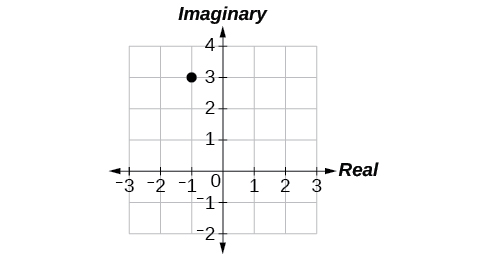## Parametric Equations

For the following exercises, eliminate the parameter $\text{\hspace{0.17em}}t\text{\hspace{0.17em}}$ to rewrite the parametric equation as a Cartesian equation.

$\left\{\begin{array}{l}x\left(t\right)=3t-1\hfill \\ y\left(t\right)=\sqrt{t}\hfill \end{array}$

${x}^{2}+\frac{1}{2}y=1$

Parameterize (write a parametric equation for) each Cartesian equation by using $\text{\hspace{0.17em}}x\left(t\right)=a\mathrm{cos}\text{\hspace{0.17em}}t\text{\hspace{0.17em}}$ and $\text{\hspace{0.17em}}y\left(t\right)=b\mathrm{sin}\text{\hspace{0.17em}}t\text{\hspace{0.17em}}$ for $\text{\hspace{0.17em}}\frac{{x}^{2}}{25}+\frac{{y}^{2}}{16}=1.$

Parameterize the line from $\text{\hspace{0.17em}}\left(-2,3\right)\text{\hspace{0.17em}}$ to $\text{\hspace{0.17em}}\left(4,7\right)\text{\hspace{0.17em}}$ so that the line is at $\text{\hspace{0.17em}}\left(-2,3\right)\text{\hspace{0.17em}}$ at $\text{\hspace{0.17em}}t=0\text{\hspace{0.17em}}$ and $\text{\hspace{0.17em}}\left(4,7\right)\text{\hspace{0.17em}}$ at $\text{\hspace{0.17em}}t=1.$

$\left\{\begin{array}{l}x\left(t\right)=-2+6t\hfill \\ y\left(t\right)=3+4t\hfill \end{array}$

## Parametric Equations: Graphs

For the following exercises, make a table of values for each set of parametric equations, graph the equations, and include an orientation; then write the Cartesian equation.

$\left\{\begin{array}{l}x\left(t\right)=3{t}^{2}\hfill \\ y\left(t\right)=2t-1\hfill \end{array}$

$\left\{\begin{array}{l}x\left(t\right)={e}^{t}\hfill \\ y\left(t\right)=-2{e}^{5\text{\hspace{0.17em}}t}\hfill \end{array}$

$y=-2{x}^{5}$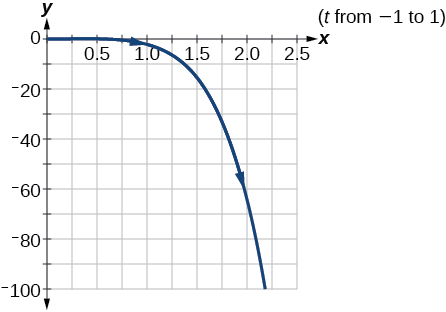$\left\{\begin{array}{l}x\left(t\right)=3\mathrm{cos}\text{\hspace{0.17em}}t\hfill \\ y\left(t\right)=2\mathrm{sin}\text{\hspace{0.17em}}t\hfill \end{array}$

A ball is launched with an initial velocity of 80 feet per second at an angle of 40° to the horizontal. The ball is released at a height of 4 feet above the ground.

1. Find the parametric equations to model the path of the ball.
2. Where is the ball after 3 seconds?
3. How long is the ball in the air?
1. $\left\{\begin{array}{l}x\left(t\right)=\left(80\mathrm{cos}\left(40°\right)\right)t\\ y\left(t\right)=-16{t}^{2}+\left(80\mathrm{sin}\left(40°\right)\right)t+4\end{array}$
2. The ball is 14 feet high and 184 feet from where it was launched.
3. 3.3 seconds

## Vectors

For the following exercises, determine whether the two vectors, $\text{\hspace{0.17em}}u\text{\hspace{0.17em}}$ and $\text{\hspace{0.17em}}v,\text{\hspace{0.17em}}$ are equal, where $\text{\hspace{0.17em}}u\text{\hspace{0.17em}}$ has an initial point $\text{\hspace{0.17em}}{P}_{1}\text{\hspace{0.17em}}$ and a terminal point $\text{\hspace{0.17em}}{P}_{2},\text{\hspace{0.17em}}$ and $\text{\hspace{0.17em}}v\text{\hspace{0.17em}}$ has an initial point $\text{\hspace{0.17em}}{P}_{3}\text{\hspace{0.17em}}$ and a terminal point $\text{\hspace{0.17em}}{P}_{4}.$

${P}_{1}=\left(-1,4\right),{P}_{2}=\left(3,1\right),{P}_{3}=\left(5,5\right)$ and $\text{\hspace{0.17em}}{P}_{4}=\left(9,2\right)$

${P}_{1}=\left(6,11\right),{P}_{2}=\left(-2,8\right),{P}_{3}=\left(0,-1\right)\text{\hspace{0.17em}}$ and $\text{\hspace{0.17em}}{P}_{4}=\left(-8,2\right)$

not equal

For the following exercises, use the vectors $\text{\hspace{0.17em}}u=2i-j\text{,}v=4i-3j\text{,}\text{\hspace{0.17em}}$ and $\text{\hspace{0.17em}}w=-2i+5j\text{\hspace{0.17em}}$ to evaluate the expression.

u v

2 v u + w

4 i

For the following exercises, find a unit vector in the same direction as the given vector.

a = 8 i − 6 j

b = −3 i j

$-\frac{3\sqrt{10}}{10}$ i $-\frac{\sqrt{10}}{10}$ j

For the following exercises, find the magnitude and direction of the vector.

$⟨6,-2⟩$

$⟨-3,-3⟩$

Magnitude: $\text{\hspace{0.17em}}3\sqrt{2},\text{\hspace{0.17em}}$ Direction: $\text{225°}$

For the following exercises, calculate $\text{\hspace{0.17em}}u\cdot v\text{.}$

u = −2 i + j and v = 3 i + 7 j

u = i + 4 j and v = 4 i + 3 j

$\text{16}$

Given v $=〈-3,4〉$ draw v , 2 v , and $\text{\hspace{0.17em}}\frac{1}{2}$ v .

Given the vectors shown in [link] , sketch u + v , u v and 3 v .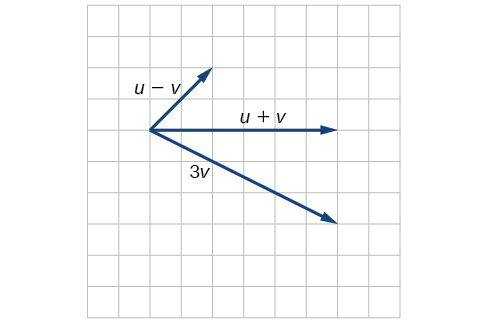Given initial point $\text{\hspace{0.17em}}{P}_{1}=\left(3,2\right)\text{\hspace{0.17em}}$ and terminal point $\text{\hspace{0.17em}}{P}_{2}=\left(-5,-1\right),\text{\hspace{0.17em}}$ write the vector $\text{\hspace{0.17em}}v\text{\hspace{0.17em}}$ in terms of $\text{\hspace{0.17em}}\text{\hspace{0.17em}}i\text{\hspace{0.17em}}\text{\hspace{0.17em}}$ and $\text{\hspace{0.17em}}j.\text{\hspace{0.17em}}$ Draw the points and the vector on the graph.

## Practice test

Assume $\text{\hspace{0.17em}}\alpha \text{\hspace{0.17em}}$ is opposite side $\text{\hspace{0.17em}}a,\beta \text{\hspace{0.17em}}$ is opposite side $\text{\hspace{0.17em}}b,\text{\hspace{0.17em}}$ and $\text{\hspace{0.17em}}\gamma \text{\hspace{0.17em}}$ is opposite side $\text{\hspace{0.17em}}c.\text{\hspace{0.17em}}$ Solve the triangle, if possible, and round each answer to the nearest tenth, given $\text{\hspace{0.17em}}\beta =68°,b=21,c=16.$

$\alpha =67.1°,\gamma =44.9°,a=20.9$

Find the area of the triangle in [link] . Round each answer to the nearest tenth.

A pilot flies in a straight path for 2 hours. He then makes a course correction, heading 15° to the right of his original course, and flies 1 hour in the new direction. If he maintains a constant speed of 575 miles per hour, how far is he from his starting position?

Convert $\text{\hspace{0.17em}}\left(2,2\right)\text{\hspace{0.17em}}$ to polar coordinates, and then plot the point.

Convert $\text{\hspace{0.17em}}\left(2,\frac{\pi }{3}\right)\text{\hspace{0.17em}}$ to rectangular coordinates.

$\left(1,\sqrt{3}\right)$

Convert the polar equation to a Cartesian equation: $\text{\hspace{0.17em}}{x}^{2}+{y}^{2}=5\mathrm{y.}$

Convert to rectangular form and graph: $r=-3\mathrm{csc}\text{\hspace{0.17em}}\theta .$

$y=-3$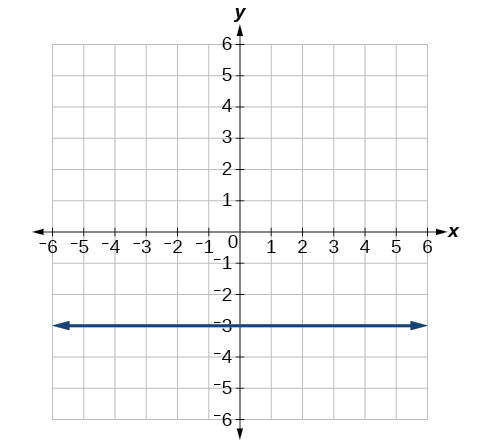Test the equation for symmetry: $\text{\hspace{0.17em}}r=-4\mathrm{sin}\left(2\theta \right).$

Graph $\text{\hspace{0.17em}}r=3+3\mathrm{cos}\text{\hspace{0.17em}}\theta .$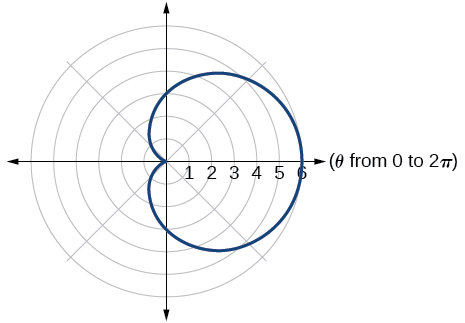Graph $\text{\hspace{0.17em}}r=3-5\text{sin}\text{\hspace{0.17em}}\theta .$

Find the absolute value of the complex number $5-9i.$

$\sqrt{106}$

Write the complex number in polar form: $\text{\hspace{0.17em}}4+i\text{.}$

Convert the complex number from polar to rectangular form: $\text{\hspace{0.17em}}z=5\text{cis}\left(\frac{2\pi }{3}\right).$

$\frac{-5}{2}+i\frac{5\sqrt{3}}{2}$

Given $\text{\hspace{0.17em}}{z}_{1}=8\mathrm{cis}\left(36°\right)\text{\hspace{0.17em}}$ and $\text{\hspace{0.17em}}{z}_{2}=2\mathrm{cis}\left(15°\right),$ evaluate each expression.

${z}_{1}{z}_{2}$

$\frac{{z}_{1}}{{z}_{2}}$

$4\mathrm{cis}\left(21°\right)$

${\left({z}_{2}\right)}^{3}$

$\sqrt{{z}_{1}}$

$2\sqrt{2}\mathrm{cis}\left(18°\right),2\sqrt{2}\mathrm{cis}\left(198°\right)$

Plot the complex number $\text{\hspace{0.17em}}-5-i\text{\hspace{0.17em}}$ in the complex plane.

Eliminate the parameter $\text{\hspace{0.17em}}t\text{\hspace{0.17em}}$ to rewrite the following parametric equations as a Cartesian equation: $\text{\hspace{0.17em}}\left\{\begin{array}{l}x\left(t\right)=t+1\hfill \\ y\left(t\right)=2{t}^{2}\hfill \end{array}.$

$y=2{\left(x-1\right)}^{2}$

Parameterize (write a parametric equation for) the following Cartesian equation by using $\text{\hspace{0.17em}}x\left(t\right)=a\mathrm{cos}\text{\hspace{0.17em}}t\text{\hspace{0.17em}}$ and $\text{\hspace{0.17em}}y\left(t\right)=b\mathrm{sin}\text{\hspace{0.17em}}t:$ $\frac{{x}^{2}}{36}+\frac{{y}^{2}}{100}=1.$

Graph the set of parametric equations and find the Cartesian equation: $\text{\hspace{0.17em}}\left\{\begin{array}{l}x\left(t\right)=-2\mathrm{sin}\text{\hspace{0.17em}}t\hfill \\ y\left(t\right)=5\mathrm{cos}\text{\hspace{0.17em}}t\hfill \end{array}.$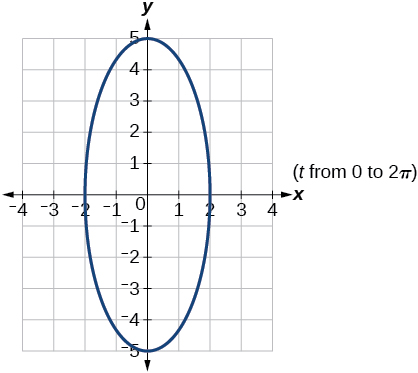A ball is launched with an initial velocity of 95 feet per second at an angle of 52° to the horizontal. The ball is released at a height of 3.5 feet above the ground.

1. Find the parametric equations to model the path of the ball.
2. Where is the ball after 2 seconds?
3. How long is the ball in the air?

For the following exercises, use the vectors u = i − 3 j and v = 2 i + 3 j .

Find 2 u − 3 v .

−4 i − 15 j

Calculate $\text{\hspace{0.17em}}u\cdot v.$

Find a unit vector in the same direction as $\text{\hspace{0.17em}}v.$

$\frac{2\sqrt{13}}{13}i+\frac{3\sqrt{13}}{13}j$

Given vector $\text{\hspace{0.17em}}v\text{\hspace{0.17em}}$ has an initial point $\text{\hspace{0.17em}}{P}_{1}=\left(2,2\right)\text{\hspace{0.17em}}$ and terminal point $\text{\hspace{0.17em}}{P}_{2}=\left(-1,0\right),\text{\hspace{0.17em}}$ write the vector $\text{\hspace{0.17em}}v\text{\hspace{0.17em}}$ in terms of $\text{\hspace{0.17em}}i\text{\hspace{0.17em}}$ and $\text{\hspace{0.17em}}j.\text{\hspace{0.17em}}$ On the graph, draw $\text{\hspace{0.17em}}v,\text{\hspace{0.17em}}$ and $\text{\hspace{0.17em}}-v.\text{\hspace{0.17em}}$

stock therom F=(x2+y2) i-2xy J jaha x=a y=o y=b
root under 3-root under 2 by 5 y square
The sum of the first n terms of a certain series is 2^n-1, Show that , this series is Geometric and Find the formula of the n^th
cosA\1+sinA=secA-tanA
why two x + seven is equal to nineteen.
The numbers cannot be combined with the x
Othman
2x + 7 =19
humberto
2x +7=19. 2x=19 - 7 2x=12 x=6
Yvonne
because x is 6
SAIDI
what is the best practice that will address the issue on this topic? anyone who can help me. i'm working on my action research.
simplify each radical by removing as many factors as possible (a) √75
how is infinity bidder from undefined?
what is the value of x in 4x-2+3
give the complete question
Shanky
4x=3-2 4x=1 x=1+4 x=5 5x
Olaiya
hi can you give another equation I'd like to solve it
Daniel
what is the value of x in 4x-2+3
Olaiya
if 4x-2+3 = 0 then 4x = 2-3 4x = -1 x = -(1÷4) is the answer.
Jacob
4x-2+3 4x=-3+2 4×=-1 4×/4=-1/4
LUTHO
then x=-1/4
LUTHO
4x-2+3 4x=-3+2 4x=-1 4x÷4=-1÷4 x=-1÷4
LUTHO
A research student is working with a culture of bacteria that doubles in size every twenty minutes. The initial population count was  1350  bacteria. Rounding to five significant digits, write an exponential equation representing this situation. To the nearest whole number, what is the population size after  3  hours?
v=lbh calculate the volume if i.l=5cm, b=2cm ,h=3cm
Need help with math
Peya
can you help me on this topic of Geometry if l help you
litshani
( cosec Q _ cot Q ) whole spuare = 1_cosQ / 1+cosQ
A guy wire for a suspension bridge runs from the ground diagonally to the top of the closest pylon to make a triangle. We can use the Pythagorean Theorem to find the length of guy wire needed. The square of the distance between the wire on the ground and the pylon on the ground is 90,000 feet. The square of the height of the pylon is 160,000 feet. So, the length of the guy wire can be found by evaluating √(90000+160000). What is the length of the guy wire?
the indicated sum of a sequence is known as
how do I attempted a trig number as a starterByByBy Janet ForresterBy Edward BitonBy Anonymous UserBy OpenStaxBy OpenStaxBy Madison ChristianBy Anh DaoBy RhodesBy JavaChamp TeamBy Briana Hamilton